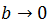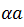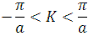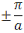# The Kronig-Penney Model - Engineering Physics

The Kronig-Penney Model

Kronig and Penney examined the behavior of electrons in a periodic potential by considering a relatively simple and one-dimensional model. It is assumed that the potential energy of an electron has the shape of a square well as shown in fig. The period of potential is (a+b)

This type of periodic potential is an approximation of the actual picture.

Now Schrodinger wave eq. for the two regions can be written as,

And

It is assumed that E the energy of an electron is smaller thanSo eq. (1) and (2) become

Since the potential is periodic, so the solution of eq. (4) and (5) must be of the form of Bloch functions i.e.

is the periodic function x with period (a+b)

And

Or

Putting eq. (6) and (7) in eq. (4) and (5), we get

represents the value of

The solutions of these equations are

Where A, B, C and D are arbitrary constants which can be determined by following boundary conditions.

Applying these boundary conditions to eqns. (10) and (11), we get

These eqns can be solved for non-zero value of A, B, C and D only if the determinant of the coefficient of A, B, C and D becomes zero.

On solving, we get

In order to make the situation more simple, Kronig and Penney considered the height of the potential barrier is very large. i.eand simultaneously the width of the barrierremain finite.

Under these circumstances, eq. (14) becomes

This eq. can be written more simply as

of the potential barrier. Large value of P means that given electron is more strongly bound to a particular potential well.

Now R.H.S of eq. (1.72) can assume values between +1 and -1 and hence only those value ofare allowed which make L.H.S of this eq. lie between +1

Fig shows left hand side of this equation as a function ofis proportional to energy E, hence abscissa gives a measure of energy.

The conclusions exclude from the above figure are given below.

1. The energy spectrum of the electrons consists of energy bands allowed and forbidden.

2. The width of the allowed energy region or band increases with increasing values of  or energy.

, the allowed region becomes infinitely narrow.

This is the result we obtain for a particle in a box of atomic dimensions with a constant potential i.e. electron tightly bound and tunneling through the barriers becomes improbable. This shows the case of an insulator.

This represents the energy of a completely free electron for which any energy value is possible. This shows the case of the conductor.

4. E-K diagram

of eq. (15) is an even periodic function and its value does not change whether Ka is positive or negative. As a result, the total energy E of the electron is an even periodic function of K. This periodic repetition of energy is shown in fig. A. This may be considered as obtained by the repetition of the regionthis is the first Brillouin zone. This representation is known as the periodic zone scheme.

However, there are two other schemes, the reduced zone scheme, and the extended zone scheme. These are shown in figures below-

We find that discontinuities occur at

These K value define the boundaries of the first, second and third Brillouin zones. If we consider a line representing K values, divided into energy discontinuities into segments of lengthas shown in Fig. , these line segments are called Brillouin zones.

1.2.3.4.